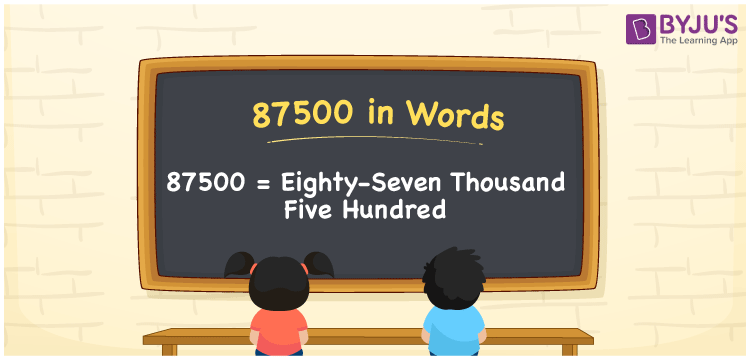# 87500 in Words

87500 in words can be written as Eighty-Seven Thousand Five Hundred. The name of 87500 is written with the help of the place values like units, tens, hundreds, thousands and ten thousands. If you spend Rs. 87500 to purchase an electric bike, then you can say that “I bought an electric bike for Eighty-Seven Thousand Five Hundred Rupees”. Here numbers in words can be written using the English alphabet. So, the number 87500 can be read as “Eighty-Seven Thousand Five Hundred” in words.

 87500 in words Eighty-Seven Thousand Five Hundred Eighty-Seven Thousand Five Hundred in Numbers 87500

## 87500 in English Words## How to Write 87500 in Words?

The place value of each digit should be known to write the chart. The place value chart of 87500 is given below.

 Ten Thousands Thousands Hundreds Tens Ones 8 7 5 0 0

The expanded form of 87500 is provided below:

8 x Ten Thousand + 7 x Thousand + 5 x Hundred + 0 × Ten + 0 × One

= 8 x 10000 + 7 x 1000 + 5 x 100 + 0 x 10 + 0 x 1

= 80000 + 7000 + 500

= 87500

= Eighty-Seven Thousand Five Hundred

Therefore, 87500 in words is written as Eighty-Seven Thousand Five Hundred.

87500 is a natural number that precedes 87501 and succeeds 87499.

87500 in words – Eighty-Seven Thousand Five Hundred

Is 87500 an odd number? – No

Is 87500 an even number? – Yes

Is 87500 a perfect square number? – No

Is 87500 a perfect cube number? – No

Is 87500 a prime number? – No

Is 87500 a composite number? – Yes

## Frequently Asked Questions on 87500 in Words

Q1

### How do you write the number 87500 in words?

87500 in words is Eighty-Seven Thousand Five Hundred.
Q2

### Find the value of 88000 minus 500.

The value of 88000 minus 500 is 87500. Therefore, 87500 in words is written as Eighty-Seven Thousand Five Hundred.
Q3

### Is 87500 a perfect square?

No, 87500 is not a perfect square as it cannot be expressed as the product of two integers which are equal.